# Word Break Problem

Given a dictionary of words and a string of characters, find if the string of characters can be broken into individual valid words from the dictionary.
Example:
Dictionary: arrays, dynamic, heaps, IDeserve, learn, learning, linked, list, platform, programming, stacks, trees
String    : IDeservelearningplatform
Output   : true
Because the string can be broken into valid words: IDeserve learning platform

## Algorithm/Insights

The given problem can be solved by using Dynamic Programming as described below:
1. Create a temporary boolean array validWords[] defined as:
validWords[i]
= true, if input substring from 0 to i forms valid words string
= false, otherwise
2. For i = 0 to input.length,
a. If input substring from 0...i is present in the dictionary, then set validWords[i] = true
b. If validWords[i] == true, from j = i+1 to n-1, check if substring from i+1 to j, for all values of j (= i+1 to n-1), is present in the dictionary and set validWords[j] to true if found in the dictionary.
3. When we reach the end of the string, if validWords[n-1] is true, then return true else return false.

Example:
Consider the dictionary: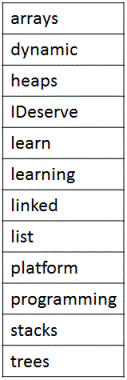Input string:
IDeservelearningplatform

Temporary array validWords[] initialized to false (represented by F in the image).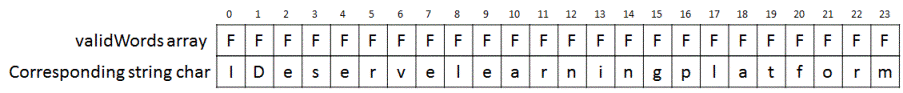i = 0:
Is input substring from 0...i (= I) present in the dictionary? ✖

i = 1:
Is input substring from 0...i (= ID) present in the dictionary? ✖

i = 2:
Is input substring from 0...i (= IDe) present in the dictionary? ✖

Proceeding till i = 6:
Is input substring from 0...i (= IDeserv) present in the dictionary? ✖

i = 7:
Is input substring from 0...i (= IDeserve) present in the dictionary? ✔
Set validWords[i] = true (represented by T in the image)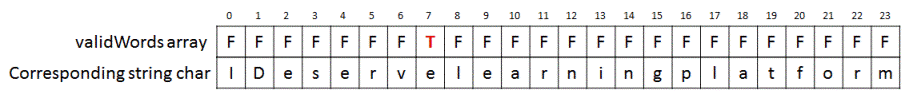Next, starting from j = i+1 to n-1, on checking for all substrings from i+1 to j, we see that 'learn' (ending at j=12) and 'learning' (ending at 15) form valid dictionary words.
So we set, validWords = true and validWords = true.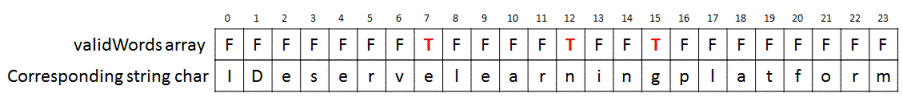Since, we have not reached the end of the input string, we proceed with next steps.

i = 8:
Is input substring from 0...i (= IDeservel) present in the dictionary? ✖

Proceeding till i = 11:
Is input substring from 0...i (= IDeservelear) present in the dictionary? ✖

i = 12: input substring = IDeservelearn
Next, starting from j = i+1 to n-1, on checking for all substrings from i+1 to j, we see that there is no valid dictionary word found.

Proceeding like before for i = 13 and 14:
Is input substring from 0...i (= IDeservelearni) present in the dictionary? ✖
Is input substring from 0...i (= IDeservelearnin) present in the dictionary? ✖

i = 15: input substring = IDeservelearning
Next, starting from j = i+1 to n-1, on checking for all substrings from i+1 to j, we see that 'platform' (ending at 23) is a valid dictionary word.
So, we set validWords = true.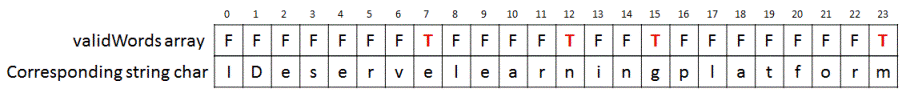Since, we have reached end of string and validWords[n-1] = validWords = true, hence we return true.

## Code Snippet

```
package com.ideserve.questions.saurabh;

import java.util.Arrays;
import java.util.HashSet;
import java.util.Set;

/**
* <b>IDeserve <br>
* Given a dictionary of words and a string of characters, find if the string of
* characters can be broken into individual valid words from the dictionary.
*
* @author Saurabh
*/
public class WordBreakProblem {

private static final Set<String> dictionary = new HashSet<String>(Arrays.asList("arrays", "dynamic", "heaps", "IDeserve", "learn", "learning", "linked", "list", "platform", "programming", "stacks", "trees"));

public static boolean hasValidWords(String words) {

// Empty string
if(words == null || words.length() == 0) {
return true;
}

int n = words.length();
boolean[] validWords = new boolean[n];
for (int i = 0; i < n; i++) {
if (dictionary.contains(words.substring(0, i + 1))) {
validWords[i] = true;
}
if (validWords[i] == true && (i == n - 1))
return true;
if (validWords[i] == true) {
for (int j = i + 1; j < n; j++) {
if (dictionary.contains(words.substring(i + 1, j + 1))) {
validWords[j] = true;
}
if (j == n - 1 && validWords[j] == true) {
return true;
}
}
}
}
return false;
}

public static void main(String[] args) {
if (hasValidWords("IDeservelearningplatform"))
System.out.println("true");
else
System.out.println("false");
}
}
```

## Order of the Algorithm

Time Complexity is O(n^2) in worst case
Space Complexity is O(n)